# ADO.NET DataSet using SQL Server

## ADO.NET DataSet using SQL Server in C#

In this article, I am going to discuss ADO.NET DataSet using SQL Server in C# with Examples. Please read our previous article where we discussed the basics of ADO.NET DataSet and the different constructors, properties, and methods of DataSet Class. At the end of this article, you will understand the following pointers.

1. What is DataSet in C#?
2. How to use ADO.NET DataSet with SQL Server Database?
3. Example to Understand ADO.NET DataSet using SQL Server Database?
4. DataSet with Multiple Database Tables using SQL Server.
5. How to Set the Data Table Name Explicitly in ADO.NET DataSet?
6. Example to understand Copy, Clone, and Clear Methods of DataSet Object in C#
7. How to Remove a DataTable from ADO.NET DataSet in C#?
##### What is DataSet in C#?

The ADO.NET DataSet is a collection of data tables that contains the relational data in memory in tabular format. It does not require an open or active connection to the database in order to fetch the data. That means the DataSet is based on the disconnected architecture. This is the reason why it is used to fetch the data without interacting with any data source. The ADO.NET DataSet class belongs to the System.Data namespace.

##### How to use ADO.NET DataSet using SQL Server Database?

Let us understand how to use DataSet in C# to fetch and store the data from the SQL Server Database with an example. We are going to use the following Customers and Orders tables to understand the ADO.NET DataSet.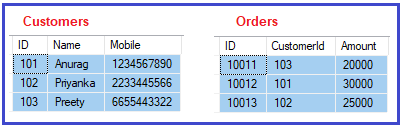Please use the below SQL Script to create a database and tables and populate the Customers and Orders tables with the required test data.

```CREATE DATABASE ShoppingCartDB;
GO

USE ShoppingCartDB;
GO

CREATE TABLE Customers(
ID INT PRIMARY KEY,
Name VARCHAR(100),
Mobile VARCHAR(50)
)
GO

INSERT INTO Customers VALUES (101, 'Anurag', '1234567890')
INSERT INTO Customers VALUES (102, 'Priyanka', '2233445566')
INSERT INTO Customers VALUES (103, 'Preety', '6655443322')
GO

CREATE TABLE Orders(
ID INT PRIMARY KEY,
CustomerId INT,
Amount INT
)
GO

INSERT INTO Orders VALUES (10011, 103, 20000)
INSERT INTO Orders VALUES (10012, 101, 30000)
INSERT INTO Orders VALUES (10013, 102, 25000)
GO```
##### Example to Understand ADO.NET DataSet using SQL Server Database in C#:

Our business requirement is to fetch all the data from the Customers table and then need to display it on the console. The following example exactly does the same using DataSet. In the below example, we created an instance of the DataSet and then fill the dataset using the Fill method of the data adapter object. The following example is self-explained, so please go through the comment lines.

```using System;
using System.Data;
using System.Data.SqlClient;

{
class Program
{
static void Main(string[] args)
{
try
{
string ConnectionString = @"data source=LAPTOP-ICA2LCQL\SQLEXPRESS; database=ShoppingCartDB; integrated security=SSPI";
using (SqlConnection connection = new SqlConnection(ConnectionString))
{
//Create the SqlDataAdapter instance by specifying the command text and connection object

//Creating DataSet Object
DataSet dataSet = new DataSet();

//Filling the DataSet using the Fill Method of SqlDataAdapter object
//Here, we have not specified the data table name and the data table will be created at index position 0

//Iterating through the DataSet
//First fetch the Datatable from the dataset and then fetch the rows using the Rows property of Datatable
foreach (DataRow row in dataSet.Tables.Rows)
{
//Accessing the Data using the string column name as key
Console.WriteLine(row["Id"] + ",  " + row["Name"] + ",  " + row["Mobile"]);

//Accessing the Data using the integer index position as key
//Console.WriteLine(row["Id"] + ",  " + row["Name"] + ",  " + row["Mobile"]);
}
}
}
catch (Exception ex)
{
Console.WriteLine(\$"Exception Occurred: {ex.Message}");
}
}
}
}```
###### Output: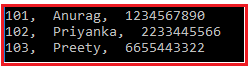By default, the dataset assigns a name to the table as Table, Table1, and Table2. So, the above example can be rewritten as shown below and it should give the same output as the previous example. As you can see, here, we are fetching the table using the name (Table).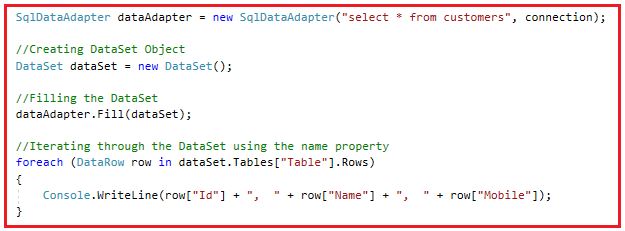##### DataSet with Multiple Database Tables using SQL Server in C#:

It is also possible that your SQL Query may return multiple tables. Let us understand this with an example. Now our business requirement is to fetch the Customers as well as Orders table data which needs to display on the Console. Here, you can access the first table from the dataset using an integral index 0 or string Table name. On the other hand, you can access the second table using the integral index 1 or the string name Table1.

###### Accessing Data table from DataSet Using Integral Index Position in C#:

The following example is self-explained, so please go through the comment lines.

```using System;
using System.Data;
using System.Data.SqlClient;
{
class Program
{
static void Main(string[] args)
{
try
{
string ConnectionString = @"data source=LAPTOP-ICA2LCQL\SQLEXPRESS; database=ShoppingCartDB; integrated security=SSPI";
using (SqlConnection connection = new SqlConnection(ConnectionString))
{
//We have written two Select Statements to return data from customers and orders table
DataSet dataSet = new DataSet();
//Data Table 1 will be customers data which is at Index Position 0
//Data Table 2 will be orders data which is at Index Position 1

// Fetching First Table Data i.e. Customers Data
Console.WriteLine("Table 1 Data");
//Accessing the Data Table from the DataSet using Integer Index Position
foreach (DataRow row in dataSet.Tables.Rows)
{
//Accessing using string column name as keys
Console.WriteLine(row["Id"] + ",  " + row["Name"] + ",  " + row["Mobile"]);

//Accessing using integer index position as keys
//Console.WriteLine(row + ",  " + row + ",  " + row);
}
Console.WriteLine();

// Fetching Second Table Data i.e. Orders Data
Console.WriteLine("Table 2 Data");
//Accessing the Data Table from the DataSet using Integer Index Position
foreach (DataRow row in dataSet.Tables.Rows)
{
//Accessing using string column name as keys
//Console.WriteLine(row["Id"] + ",  " + row["CustomerId"] + ",  " + row["Amount"]);

//Accessing using integer index position as keys
Console.WriteLine(row + ",  " + row + ",  " + row);
}
}
}
catch (Exception ex)
{
Console.WriteLine(\$"Exception Occurred: {ex.Message}");
}
}
}
}```
###### Output: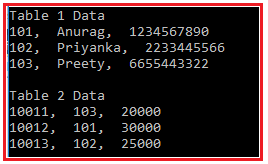###### Accessing Data table from DataSet Using Default Table Name in C#:

The following example is self-explained, so please go through the comment lines.

```using System;
using System.Data;
using System.Data.SqlClient;
{
class Program
{
static void Main(string[] args)
{
try
{
string ConnectionString = @"data source=LAPTOP-ICA2LCQL\SQLEXPRESS; database=ShoppingCartDB; integrated security=SSPI";
using (SqlConnection connection = new SqlConnection(ConnectionString))
{
//We have written two Select Statements to return data from customers and orders table
DataSet dataSet = new DataSet();
//Data Table 1 will be customers data which is at Index Position 0
//Data Table 2 will be orders data which is at Index Position 1

// Fetching First Table Data i.e. Customers Data
Console.WriteLine("Table 1 Data");
//Accessing the Data Table from the DataSet using Default Table name
//By Default, first table name is Table
foreach (DataRow row in dataSet.Tables["Table"].Rows)
{
//Accessing using string column name as keys
Console.WriteLine(row["Id"] + ",  " + row["Name"] + ",  " + row["Mobile"]);

//Accessing using integer index position as keys
//Console.WriteLine(row + ",  " + row + ",  " + row);
}
Console.WriteLine();

// Fetching Second Table Data i.e. Orders Data
Console.WriteLine("Table 2 Data");
//Accessing the Data Table from the DataSet using Default Table name
//By Default, second table name is Table1
foreach (DataRow row in dataSet.Tables["Table1"].Rows)
{
//Accessing using string column name as keys
//Console.WriteLine(row["Id"] + ",  " + row["CustomerId"] + ",  " + row["Amount"]);

//Accessing using integer index position as keys
Console.WriteLine(row + ",  " + row + ",  " + row);
}
}
}
catch (Exception ex)
{
Console.WriteLine(\$"Exception Occurred: {ex.Message}");
}
}
}
}
```

It will also give the same output as the previous example.

##### How to Set the Data Table name Explicitly in ADO.NET DataSet?

If your dataset going to contain multiple tables of data, then it is very difficult for you to identify using the integral index position or using the default table names. In such a scenario, it is always recommended to provide an explicit name for the data table. Let us understand this with an example. Now, we need to set the first table as Customers and the second table as Orders and then we need to use these custom table names to fetch the actual table data. You can set the table name using the TableName property as shown in the below image.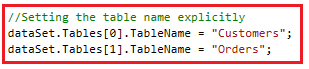The following is the complete example that uses the tableName property of the dataset object to set and get the table name. The following example code is self-explained, so please go through the comment lines.

```using System;
using System.Data;
using System.Data.SqlClient;
{
class Program
{
static void Main(string[] args)
{
try
{
string ConnectionString = @"data source=LAPTOP-ICA2LCQL\SQLEXPRESS; database=ShoppingCartDB; integrated security=SSPI";
using (SqlConnection connection = new SqlConnection(ConnectionString))
{
//We have written two Select Statements to return data from customers and orders table
DataSet dataSet = new DataSet();
//Data Table 1 will be customers data which is at Index Position 0
//Data Table 2 will be orders data which is at Index Position 1

dataSet.Tables.TableName = "Customers";
dataSet.Tables.TableName = "Orders";

// Fetching First Table Data i.e. Customers Data
Console.WriteLine("Table 1 Data");
//Accessing the Data Table from the DataSet using the Custom Table name
foreach (DataRow row in dataSet.Tables["Customers"].Rows)
{
//Accessing using string column name as keys
Console.WriteLine(row["Id"] + ",  " + row["Name"] + ",  " + row["Mobile"]);

//Accessing using integer index position as keys
//Console.WriteLine(row + ",  " + row + ",  " + row);
}
Console.WriteLine();

// Fetching Second Table Data i.e. Orders Data
Console.WriteLine("Table 2 Data");
//Accessing the Data Table from the DataSet using the Custom Table name
foreach (DataRow row in dataSet.Tables["Orders"].Rows)
{
//Accessing using string column name as keys
//Console.WriteLine(row["Id"] + ",  " + row["CustomerId"] + ",  " + row["Amount"]);

//Accessing using integer index position as keys
Console.WriteLine(row + ",  " + row + ",  " + row);
}
}
}
catch (Exception ex)
{
Console.WriteLine(\$"Exception Occurred: {ex.Message}");
}
}
}
}```
###### Output: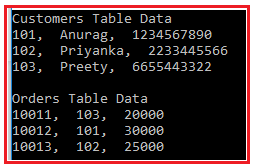Note: Once you set the custom name for a data table, then you cannot access that table using the default name. You will not get any compilation error, but at runtime, you will get the runtime error.

##### Important Methods of DataSet in C#:

Let us understand a few important methods of DataSet with examples. We are going to discuss the following three important methods of the DataSet object:

1. Copy(): Copies both the structure and data of the DataSet. That means it returns a new DataSet with the same structure (table schemas, relations, and constraints) and data as the original DataSet.
2. Clone(): Copies the structure of the DataSet, including all schemas, relations, and constraints. But does not copy any data. That means it returns a new DataSet with the same schema as the current DataSet but without the data.
3. Clear(): Clears the DataSet of any data by removing all rows in all tables.
##### Example to understand Copy, Clone, and Clear Methods of DataSet Object in C#:

The following example shows how to use the Copy, Clone, and Clear Methods of DataSet. The example code is self-explained, so please go through the comment lines.

```using System;
using System.Data;
using System.Data.SqlClient;

{
class Program
{
static void Main(string[] args)
{
try
{
string ConnectionString = @"data source=LAPTOP-ICA2LCQL\SQLEXPRESS; database=StudentDB; integrated security=SSPI";
using (SqlConnection connection = new SqlConnection(ConnectionString))
{
DataSet originalDataSet = new DataSet();
da.Fill(originalDataSet);

Console.WriteLine("Original Data Set:");
foreach (DataRow row in originalDataSet.Tables.Rows)
{
Console.WriteLine(row["Name"] + ",  " + row["Email"] + ",  " + row["Mobile"]);
}
Console.WriteLine();

Console.WriteLine("Copy Data Set:");
//Copies both the structure and data for this System.Data.DataSet.
DataSet copyDataSet = originalDataSet.Copy();
if (copyDataSet.Tables != null)
{
foreach (DataRow row in copyDataSet.Tables.Rows)
{
Console.WriteLine(row["Name"] + ",  " + row["Email"] + ",  " + row["Mobile"]);
}
}
Console.WriteLine();

Console.WriteLine("Clone Data Set");
// Copies the structure of the DataSet, including all DataTable
// schemas, relations, and constraints. Does not copy any data.
DataSet cloneDataSet = originalDataSet.Clone();
if (cloneDataSet.Tables.Rows.Count > 0)
{
foreach (DataRow row in cloneDataSet.Tables.Rows)
{
Console.WriteLine(row["Name"] + ",  " + row["Email"] + ",  " + row["Mobile"]);
}
}
else
{
Console.WriteLine("Clone Data Set is Empty");
Console.WriteLine("Adding Data to Clone Data Set Table");

foreach (DataRow row in cloneDataSet.Tables.Rows)
{
Console.WriteLine(row["Name"] + ",  " + row["Email"] + ",  " + row["Mobile"]);
}
}

Console.WriteLine();
//Clears the DataSet of any data by removing all rows in all tables.
copyDataSet.Clear();
if(copyDataSet.Tables.Rows.Count > 0)
{
foreach (DataRow row in copyDataSet.Tables.Rows)
{
Console.WriteLine(row["Name"] + ",  " + row["Email"] + ",  " + row["Mobile"]);
}
}
else
{
Console.WriteLine("After Clear No Data is Their...");
}
}
}
catch (Exception ex)
{
Console.WriteLine(\$"Exception Occurred: {ex.Message}");
}

}
}
}
```
###### Output: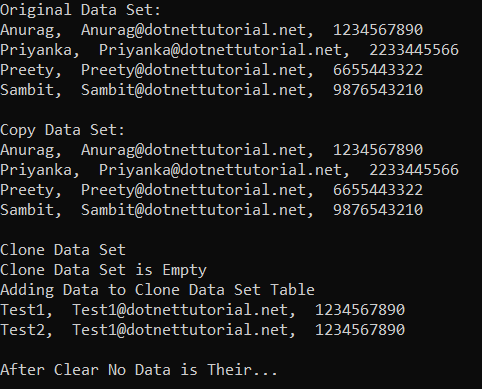##### How to Remove a DataTable from ADO.NET DataSet in C#?

Now, suppose you have a DataSet object which contains multiple data tables. If you initialize the data set object with null then all the data tables are going to be dropped. That means you cannot access the data tables anymore. But what is our requirement, instead of deleting all the data tables, I want to delete a specific data table and I still want to access other data tables. For this, we need to use the following Remove method.

1. Remove(DataTable table): This method removes the specified DataTable object from the collection. Here, the parameter table specifies the DataTable to remove.

Note: We need to call the above Remove method on the Tables collection property of the DataSet object. Further to avoid the Runtime exception, first you need to check whether the DataSet contains the data table which you are trying to remove as well as you also need to check if the DataTable can be removed from DataSet.

##### Example to Remove a DataTable from a Dataset in C#:

The following example shows how to remove a data table from a DataSet. The following example code is self-explained, so please go through the comment lines.

```using System;
using System.Data;
using System.Data.SqlClient;
{
class Program
{
static void Main(string[] args)
{
try
{
string ConnectionString = @"data source=LAPTOP-ICA2LCQL\SQLEXPRESS; database=ShoppingCartDB; integrated security=SSPI";
using (SqlConnection connection = new SqlConnection(ConnectionString))
{
//We have written two Select Statements to return data from customers and orders table
DataSet dataSet = new DataSet();
//Data Table 1 will be customers data which is at Index Position 0
//Data Table 2 will be orders data which is at Index Position 1

dataSet.Tables.TableName = "Customers";
dataSet.Tables.TableName = "Orders";

// Fetching First Table Data i.e. Customers Data
Console.WriteLine("Customers Data");
//Accessing the Data Table from the DataSet using the Custom Table name
foreach (DataRow row in dataSet.Tables["Customers"].Rows)
{
//Accessing using string column name as keys
Console.WriteLine(row["Id"] + ",  " + row["Name"] + ",  " + row["Mobile"]);
}
Console.WriteLine();

// Fetching Second Table Data i.e. Orders Data
Console.WriteLine("Orders Data");
//Accessing the Data Table from the DataSet using the Custom Table name
foreach (DataRow row in dataSet.Tables["Orders"].Rows)
{
//Accessing using integer index position as keys
Console.WriteLine(row + ",  " + row + ",  " + row);
}

Console.WriteLine();
//Now, we want to delete the Orders data table from the DataSet
if (dataSet.Tables.Contains("Orders") && dataSet.Tables.CanRemove(dataSet.Tables["Orders"]))
{
Console.WriteLine("Deleting Orders Data Table..");
dataSet.Tables.Remove(dataSet.Tables["Orders"]);
//dataSet.Tables.Remove(dataSet.Tables);
}

//Now check whether the DataTable exists or not
if (dataSet.Tables.Contains("Orders"))
{
Console.WriteLine("Orders Data Table Exits");
}
else
{
Console.WriteLine("Orders Data Table Not Exits Anymore");
}
}
}
catch (Exception ex)
{
Console.WriteLine(\$"Exception Occurred: {ex.Message}");
}
}
}
}
```
###### Output: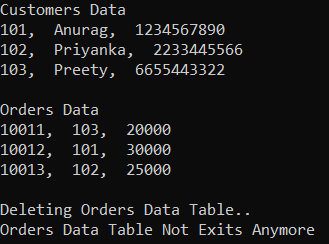1.